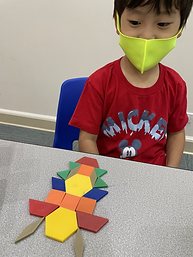## Ms. Kayla

### Target 1​

###### Lesson Type:

Continuation

Number Operation

:

Computation

Combine given quantities by using counting strategies.

###### 1:

Understand that combining numbers creates a larger quantity.

###### 2:

Represent “putting together” with objects, fingers, drawings, etc.

Kindergarden

###### Vocabulary:

Balance, Straight, Equal

Activities:

1. Students explored number equivalance with a number balance and addition. Students would put a weight on a number and try different combinations to make the balance balanaced. Students noticed when there was more on one side that would shift the balance to one side.

2. Using two weights on one side, students tried to figure out placement of one weight to make the balance straight or equal. For example, weights were placed on 3 and 7 on one side of the balance then where could the third weight be placed to make the balance straight or equal. The third weight should be placed on 10.### Home Exploration

###### Guiding Questions:## Absent Students:

### Target 2

:

###### 1:

Understand that “taking away” makes a smaller quantity.

###### 2:

Represent “taking away” with objects, fingers, drawings, etc.

Kindergarden

###### Vocabulary:

Take Away, Counter, Count, Number

Activities:

1. Students used counters to explore the concept of subtraction. Students were given 10 counters. Students counted out the counters and were directed to flip over a specific number of counters to show either yellow or red. Students would then determine the number of red or yellow showing to make 10 by counting each color separately.### Home Exploration

###### Guiding Questions:### Target 3

:

###### 1:

Compose larger shapes from smaller shapes (two small triangles can make a rectangle).

Kindergarden

###### Vocabulary:

Shape, Symmetry,

Activities:

1. Students used pattern blocks to create symmetrical images with a line of symmetry. As students constructed their images, they discovered smaller shapes could be used to create bigger shapes.### Home Exploration